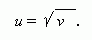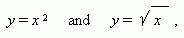# Inverse function

If  to change an argument and a function by roles, then x will be a function of y . In this case we say about a new function, called an inverse function . Assume, we have a function:

v = u 2 ,

where u is an argument and v is a function. If to change them by roles, we’ll receive u as a function of v :If  to mark in both of the functions an argument as x and a function as y , then we have two functions:each of which is an inverse one to another.

E x a m p l e s . These functions are inverse one to another:

1)  sin x and  Arcsin x ,  because if y = sin x ,  then x = Arcsin y ;
2)  cos x and  Arccos x ,  because if y = cos x ,  then x = Arccos y ;
3)  tan x and  Arctan x ,  because if y = tan x ,  then x = Arctan y ;
4) e x and  ln x ,  because if y = e x ,  then x = ln y.